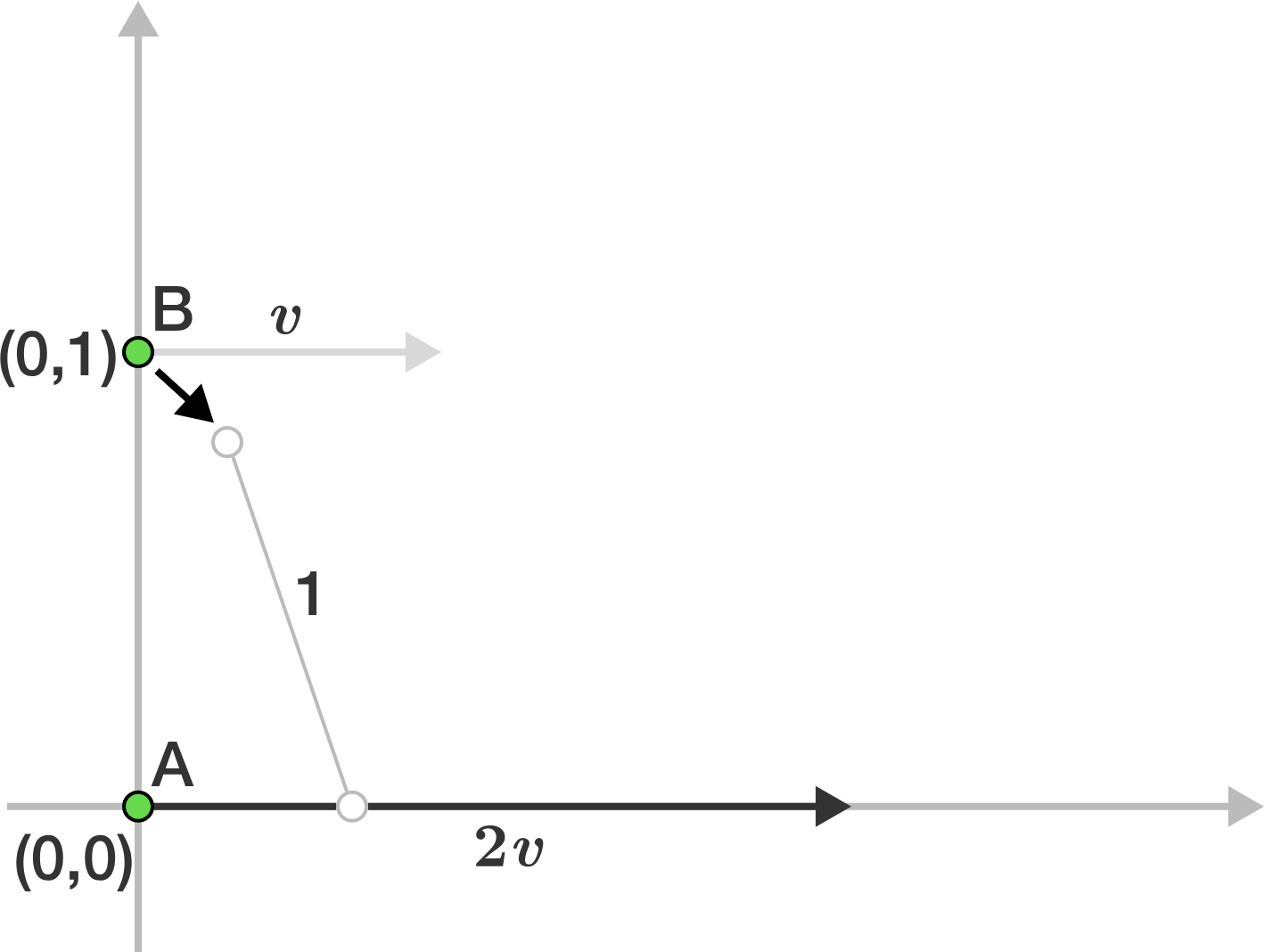# Issac's points

Geometry Level 3There exists $2$ points on Cartesian coordinate. Point $B$ has coordinates $(0,1)$. Point $A$ is on the origin $(0,0)$.

Point $A$ and $B$ always has a constant distance of $1$ from each other.

Point $B$ moves with a horizontal velocity (parallel to the x-axis) of $v.$

Point $A$ moves with a horizontal velocity of $2v.$

Point $A$'s vertical velocity (parallel to the y-axis) is $0$ while Point $B$ is allowed to move vertically in order to keep the constant distance of $1$.

All this movement is happening in the first quadrant.

Let $P$ be the area made by the figure defined by: the $x$ axis, the $y$ axis, and the path traveled by point $B$ up to where it meets the $x$ axis. Find $\left\lfloor 1000P \right\rfloor .$

After you solve this, you might want to try a continuation of this problem.

Try my Other Problems

×